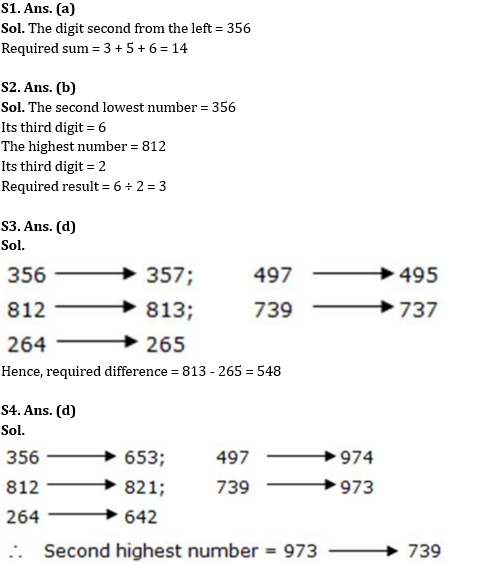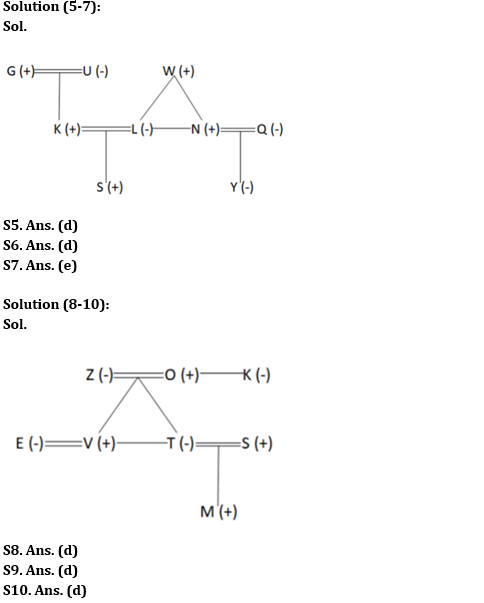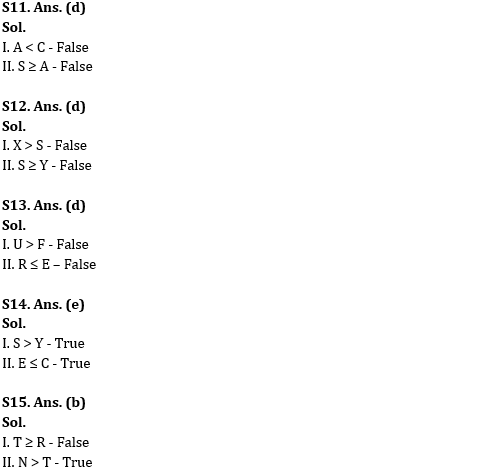Latest Banking jobs   »

# Reasoning Ability Quiz For SBI Clerk Prelims 2022- 19th May

Directions (1-4): The following questions are based on the five three-digit numbers given below.

356   497   812   739   264

Q1. If all the numbers are arranged in ascending order from left to right, which of the following will be the sum of all the three digits of the number which is second from the left of the new arrangement thus formed?
(a) 14
(b) 19
(c) 20
(d) 11
(e) 12

Q2. What will be the resultant if the third digit of the second lowest number is divided by the third digit of the highest number?
(a) 2
(b) 3
(c) 4
(d) 5
(e) 2.6

Q3. If ‘2’ is subtracted from the last digit of every odd number and 1 is added to the last digit of every even number, what will be the difference between the highest and the lowest numbers thus formed?
(a) 381
(b) 456
(c) 318
(d) 548
(e) 242

Q4. If all the digits in each of the numbers are arranged in descending order within the number, which of the following will form the second highest number in the new arrangement of numbers?
(a) 356
(b) 497
(c) 812
(d) 739
(e) 264

Directions (5-7): Study the information carefully and answer the questions accordingly.
N has no son. L is the daughter-in-law of G. N is married to Q. L is the daughter of W. W has two children. S is the grandson of U. G has only one son. The number of males is more than the number of females. U and W are not a couple. Q is the mother of Y. K is the only child of G. N and L are siblings. G is not the grandmother of S.

Q5. Who is the mother of S?
(a) Y
(b) The daughter of U
(c) The wife of N
(d) L
(e) None of these

Q6. How W is related to Q?
(a) Grandfather
(b) Father
(c) Mother-in-law
(d) Father-in-law
(e) None of these

Q7. Four from the following are similar in a certain way and forms a group. Find out the one, which does not belong to that group.
(a) L, N
(b) S, Y
(c) K, U
(d) G, Q
(e) W, S

Directions (8-10): Study the data carefully and answer the questions accordingly.
There are eight members in a three-generation family with three married couples. K is the sister-in-law of Z who has no siblings. V is the uncle of M, who is the grandson of O. S is the son-in-law of Z, who is the wife of O. E is the sister-in-law of T, who is the niece of K. V and T are siblings.

Q8. How S is related to M?
(a) Nephew
(b) Brother
(c) Uncle
(d) Father
(e) None of these

Q9. Who among the following is a couple?
(a) T, M
(b) E, S
(c) Z, V
(d) V, E
(e) None of these

Q10. Who is the daughter of O?
(a) The wife of V
(b) K
(c) The aunt of M
(d) T
(e) None is correct

Directions (11-15): In the following questions assuming the given statements to be True, find which of the conclusion among given conclusions is / are definitely true and then give your answers accordingly.

Q11. Statements: B< Y <A ≥ Q = N >C; S = T ≥ G = V >B
Conclusions:
I. A<C
II. S ≥ A
(a) Only I is true
(b) Only II is true
(c) Either I or II is true
(d) Neither I nor II is true
(e) Both I and II are true

Q12. Statements: Y ≥ M = K< B = G <S ≥ V > L; X > Z > M
Conclusions:
I. X >S
II. S ≥ Y
(a) Only I is true
(b) Only II is true
(c) Either I or II is true
(d) Neither I nor II is true
(e) Both I and II are true

Q13. Statements: R< Q ≥ G; G ≥ F ≥ E; C ≤ R; C > U
Conclusions:
I. U > F
II. R ≤ E
(a) Only I is true
(b) Only II is true
(c) Either I or II is true
(d) Neither I nor II is true
(e) Both I and II are true

Q14. Statement: S> A ≥ E ≤ X ≤ C; A = Y
Conclusions:
I. S>Y
II.E ≤ C
(a) Only I is true
(b) Only II is true
(c) Either I or II is true
(d) Neither I nor II is true
(e) Both I and II are true

Q15. Statement: N = K ≥ L ≥ P < O < U ≥ R; P >T
Conclusions:
I. T ≥ R
II. N >T
(a) Only I is true
(b) Only II is true
(c) Either I or II is true
(d) Neither I nor II is true
(e) Both I and II are true

Solutions#### Congratulations!